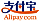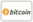Copertina di Minimax-robust estimation technique
Titolo del libro:

# Minimax-robust estimation technique

## For stationary stochastic processes

LAP LAMBERT Academic Publishing (06.08.2012 )eleggibile a buono
ISBN-13:

### 978-3-659-19817-5

ISBN-10:
365919817X
EAN:
9783659198175
Lingua del libro:
Inglese
Risvolto di copertina:
Description of methods of estimation of linear functionals of the unknown values of vector-valued stationary stochastic sequences and processes is presented. Extrapolation, interpolation and filtering problems are investigated. Two main approaches to solution of the estimation problems are developed. The first one, the spectral certainty case, is based on the assumption that matrices of spectral densities of stochastic sequences and processes are known exactly. In this case we derived formulas for calculation the spectral characteristics and mean-square errors of the optimal estimates of the functionals which determine the extrapolation, interpolation and filtering problems for stochastic sequences and processes. The second one, the case of spectral uncertainty, is based on assumption that matrices of spectral densities of the processes are not known exactly, but, instead, classes of admissible values of spectral densities are specified. These classes of densities describe different models of vector-valued stationary stochastic processes.
Casa editrice:
Sito Web:
https://www.lap-publishing.com/
Da (autore):
Mikhail Moklyachuk, Oleksandr Masyutka
Numero di pagine:
296
Pubblicato il:
06.08.2012
Giacenza di magazzino:
Disponibile
categoria:
Teoria della probabilità, processi stocastici, statistica matematica
Prezzo:
2.797,39 NT\$
Parole chiave:
Filtering, stochastic processes, interpolation, Extrapolation, optimal estimate, robust spectral characteristics, least favourable spectral densities### Categorie